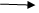Display data using entity framework model class
Ratings:
(4)
Views:739## Display data using entity framework model class

Performing the same example by writing code in model class Sol :-    Create a new view in home folder  (sample) Creating MODEL CLASS :-

• Go to solution explorer
• Go to model folder
• Double click on account model.c
• Click on + symbol of model
• Create a class with name sample model
```Public class Sample model ()
{
Public data set ds;
Public sample model ()
{
Sql connection  con = new sql connection (“”);
String s= “select * from emp details “;
Data set ds = new data set ();
Da.fill (ds,’x’);
}
}```Go to home controller .cs

```Aspired to become an ASP.net ?
Explore the post to discover the know-hows on ASP.net Training.```write the following code for action method sample ();

```Public action result sample ()
{
Models.sample1 model obj1 = new models.sample1 model ();
List <data row> r = row list <data row> ();
Foreach (Dat row x in obj1.ds.tables .Rows)
View data [‘R’] = R;
Return view ();
}
}
}```Go to sample 1 .aspx and write the following code: <% @ import name space = ‘system.data’ %> <h2>satya technologies </h2> <div> <% HTML. Begin form () ; %>Change the action name in global.aspx and run the application and check.

For indepth understanding click on